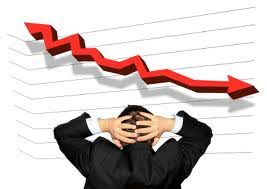# October 2017

## Probability of Negative ReturnIn our earlier article, ‘What is a Nap?’, we briefly mentioned the probability of negative return but, at that stage, we didn’t expound on what it is, how it’s calculated or how to use it in relation to horseracing results.

However, what we did say in the earlier article was that to properly analyse a series of horseracing selections, such as those supplied by a tipster, you need a large number of selections. The reason for this is that, when the number of selections is large, the distribution of horseracing data looks the normal, or Gaussian, distribution for a random variable.

The probability of negative return is a simple statistic to calculate and understand. The figure calculated represents the probability of a series of selections producing a negative return or, in other words, a level stakes loss. The higher the figure calculated the higher the probability of incurring such as loss.

In order to calculate the probability of negative return for a series of selections, you need to:

Calculate the theoretical percentage profit from each selection, to level stakes. A winner at even money would generate a profit of 100%, a winner at 2/1 would generate a profit of 200% and so on, while any loser would generate a profit of -100%.

Calculate the average, or mean, percentage profit. The easiest way to do this (and the subsequent steps in the calculation) is to create a spreadsheet in Microsoft Excel – which can be as simple as a single column containing the percentage profit for each selection – and use the AVERAGE function.

Calculate the standard deviation of the data in your spreadsheet using the STDEV function. Algebraically, standard deviation, S, is calculated according to the formula below, but if you use Microsoft Excel you don’t need to worry about the mathematics.

If your percentage profit data is contained in, say, cells A1 to A8 of your spreadsheet, simply use the STDEV function in the form STDEV(A1:A8).

Divide the result by the square root of the number of selections, using the SQRT function, to give the standard error.

Assuming that horse racing results are at least approximately normally distributed, you can use the NORMDIST function in Excel, in the form = NORMDIST (0, mean, stderr, 1), where mean and stderr are the mean percentage profit and standard error of the percentage profit, which you calculated in the steps 2. and 4. above.

The result should be a figure between 0 and 1 and, as mentioned above, the higher the figure the higher the probability of negative return and versa. A probability of 0.10 represents a 10% chance of making a loss, or a 90% chance of making a profit, from a series of selections and is typically the maximum value that you’d want to accept if you’re going to back future selections with real money.

We hope you enjoyed ‘Probability of Negative Return’ and we will be back soon with another advanced betting guide. In the meantime, we would love to hear your thoughts on ‘Probability of Negative Return’ in the comments section below.